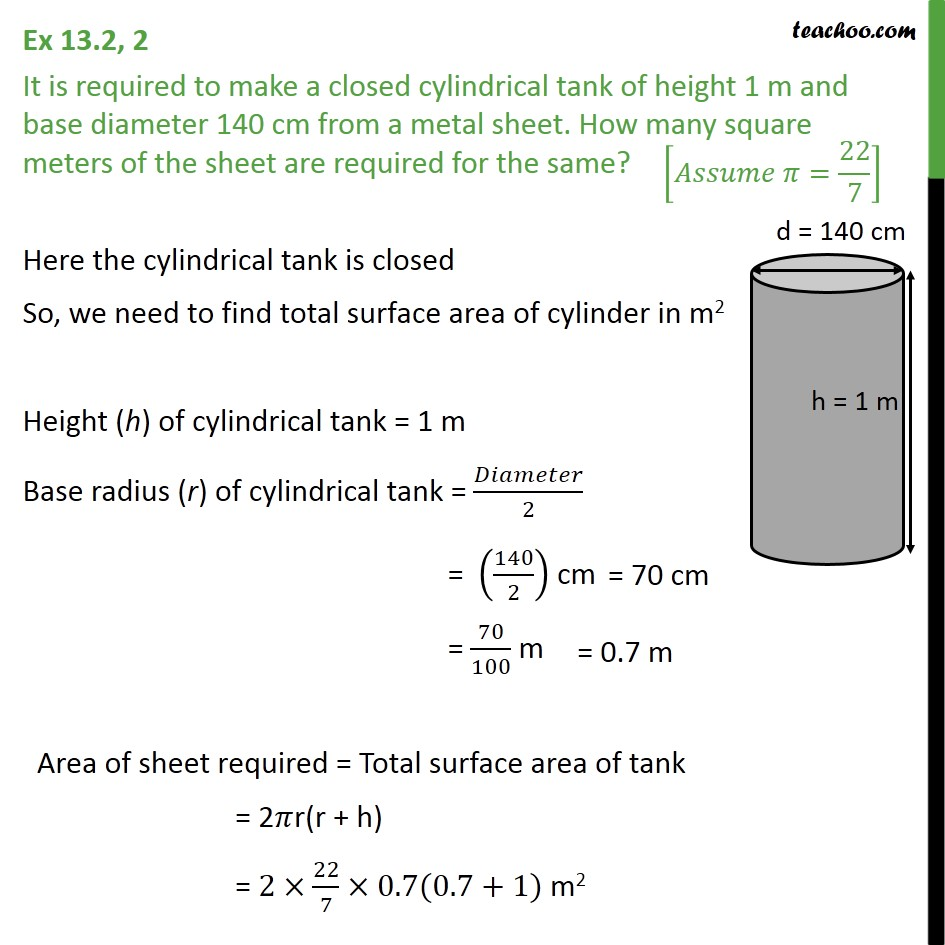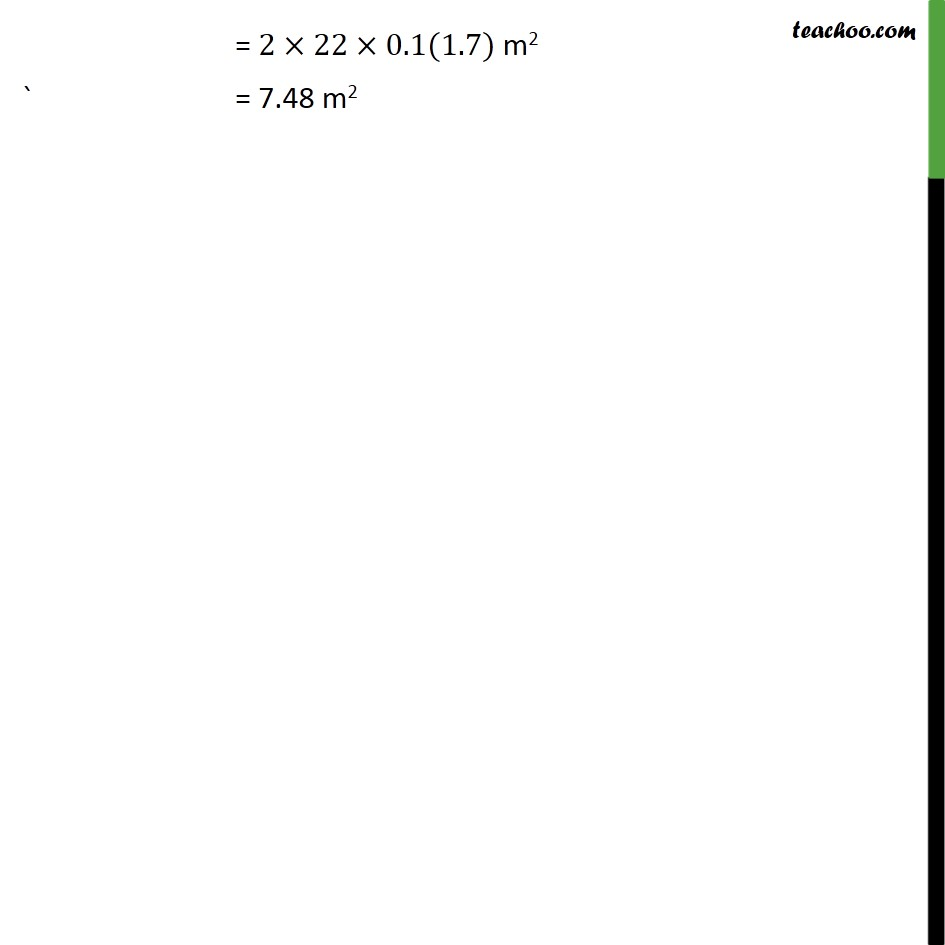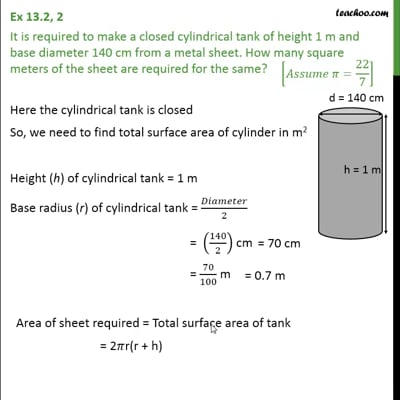Ex 13.2

Chapter 13 Class 9 Surface Areas and Volumes
Serial order wiseThis video is only available for Teachoo black users

Maths Crash Course - Live lectures + all videos + Real time Doubt solving!

### Transcript

Ex 13.2, 2 It is required to make a closed cylindrical tank of height 1 m and base diameter 140 cm from a metal sheet. How many square meters of the sheet are required for the same? Here the cylindrical tank is closed So, we need to find total surface area of cylinder in m2 Height (h) of cylindrical tank = 1 m Base radius (r) of cylindrical tank = /2 = (140/2) cm = 70/100 m Area of sheet required = Total surface area of tank = 2 r(r + h) = 2 22/7 0.7(0.7+1) m2 = 2 22 0.1(1.7) m2 ` = 7.48 m2